# Spherical Surfaces and Hat Boxes

To round off our series on round objects (see the first and second posts), let’s compute the sphere’s surface area. We can compute this in the same way we related the area and circumference of a circle two weeks ago. Approximate the surface of the sphere with lots of small triangles, and connect these to the center of the sphere to create lots of triangular pyramids. Each pyramid has volume $$\frac{1}{3}(\text{area of base})(\text{height})$$, where the heights are all nearly $$r$$ and the base areas add to approximately the surface area. By using more and smaller triangles these approximations get better and better, so the volume of the sphere is $$\frac{4}{3}\pi r^3 = \frac{1}{3}(\text{surface area})\cdot r,$$ meaning the surface area is $$4\pi r^2$$. (This and previous arguments can be made precise with the modern language of integral calculus.)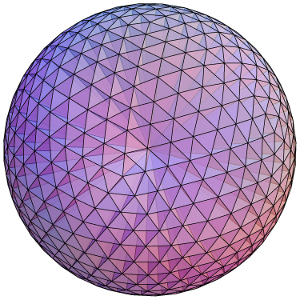Dividing a sphere into many pyramids connected to the center allows us to relate the sphere's surface area and volume.

Here’s an elegant way to rephrase this result: The surface area of a sphere is equal to the area of the curved portion of a cylinder that exactly encloses the sphere. In fact, something very surprising happens here!:

Archimedes’ Hat-Box Theorem: If we draw any two horizontal planes as shown below, then the portions of the sphere and the cylinder between the two planes have the same surface area.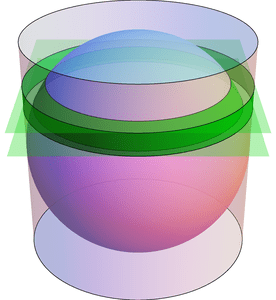Any two horizontal planes cut off a band on the sphere and another band on the enclosing cylinder. Archimedes' Hat-Box Theorem says that these bands have the same area. (The planes shown here have heights $$0.4\cdot r$$ and $$0.6\cdot r$$ above the equator.)

We can prove this with (all!) the methods in the last few posts; here’s a quick sketch. To compute the area of the “spherical band” (usually called a spherical zone), first consider the solid spherical sector formed by joining the spherical zone to the center: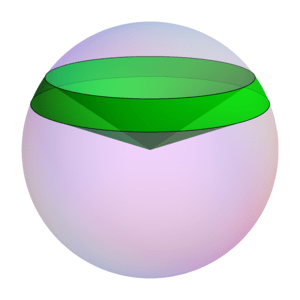The Hat-Box theorem can be proved by relating the area of the spherical zone to the volume of this spherical sector.

By dividing this into lots of triangular pyramids as we did with the sphere above, we can compute the area of the spherical zone by instead computing the sector’s volume. This volume can be computed by breaking it into three parts: two cones and the spherical segment between the two planes (on the left of the next figure). Compute the volume of the spherical segment by comparing (via Cavalieri’s Principle) to the corresponding part of the vase (from the previous post), which can be expressed with just cylinders and cones.

See if you can fill in the details!

# Slicing Spheres

Last week we saw how to compute the area of a circle from first principles. What about spheres?

To compute the volume of a sphere, let’s show that a hemisphere (with radius $$r$$) has the same volume as the vase shown in the figure below, formed by carving a cone from the circular cylinder with radius and height $$r$$. Why this shape? Here’s why: if we cut these two solids at any height $$h$$ (between 0 and $$r$$), the areas of the two slices match. Indeed, the slice—usually called cross section—of the sphere is a circle of radius $$\sqrt{r^2-h^2}$$, which has area $$\pi(r^2-h^2)$$. Similarly, the vase’s cross section is a radius $$r$$ circle with a radius $$h$$ circle cut out, so its area is $$\pi r^2-\pi h^2$$, as claimed.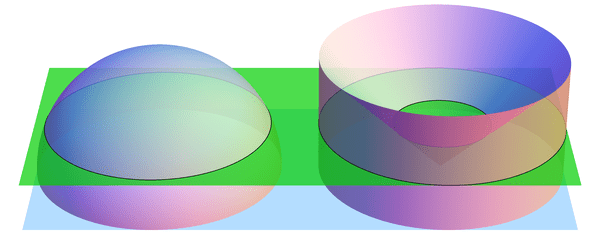When sliced by a horizontal plane at any height $$h$$, the hemisphere and vase have equal cross-sectional areas. (Shown here for $$h = 0.4\cdot r$$.) By Cavalieri's Principle, this implies that they have equal volumes.

If we imagine the hemisphere and vase as being made from lots of tiny grains of sand, then we just showed, intuitively, that the two solids have the same number of grains of sand in every layer. So there should be the same number of grains in total, i.e., the volumes should match. This intuition is exactly right:

Cavalieri’s Principle: any two shapes that have matching horizontal cross sectional areas also have the same volume.

So the volumes are indeed equal, and all that’s left is to compute the volume of the vase. But we can do this! Recall that the cone has volume $$\frac{1}{3} (\text{area of base}) (\text{height}) = \frac{1}{3}\pi r^3$$ (better yet, prove this too! Hint: use Cavalieri’s Principle again to compare to a triangular pyramid). Likewise, the cylinder has volume $$(\text{area of base}) (\text{height}) = \pi r^3$$, so the vase (and hemisphere) have volume $$\pi r^3 – \frac{1}{3} \pi r^3 = \frac{2}{3}\pi r^3$$. The volume of the whole sphere is thus $$\frac{4}{3}\pi r^3$$. Success!

The following visualization illustrates what we have shown, namely $$\text{hemisphere} + \text{cone} = \text{cylinder}.$$ The “grains of sand” in the hemisphere are being displaced horizontally by the stabbing cone, and at the end we have exactly filled the cylinder.

# Kissing Spheres

If you put a quarter flat on a table, you can surround it with six other quarters that all touch the original. Is this the best you can do, if the coins can’t overlap? Well, yes: the coins are packed as tightly as possible, so there is no room for a seventh coin to fit around the middle one. We call this the Kissing Number Problem, and it gets much more interesting if you jump from two dimensions to three: given a sphere of radius 1, how many nonoverlapping spheres (of radius 1) can you place around it such that they all “kiss” the original sphere? Said differently, what is the kissing number in 3 dimensions?

Here’s a pretty good attempt: If you put one sphere at each vertex of a cuboctahedron like this,Twelve spheres all kissing a central (blue) sphere and arranged at the vertices of a cuboctahedron.

you’ll end up with 12 that seem pretty tightly packed. In another try, you could put a sphere at each vertex of an icosahedron: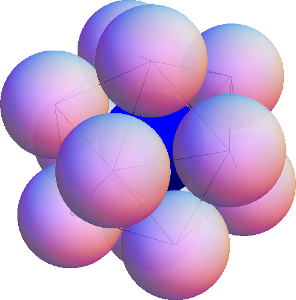Twelve spheres all kissing a central (blue) sphere and arranged at the vertices of an icosahedron.

You’ll again get 12, but this time there is noticable wiggle room to move the spheres without losing contact with the central sphere—so much wiggle room, in fact, that any two spheres can switch places! Is there enough extra space for a 13th sphere to fit? This was a topic of heated debate between Isaac Newton and David Gregory (who believed 12 and 13, respectively), but it was not resolved until much later, in 1953, in favor of Newton’s 12. The proof is highly nontrivial.

What about still higher dimensions? What is the kissing number in four dimensions? (What does a 4-dimensional sphere even look like? Perhaps a topic of a future post…) This too is known, and the answer is 24—the proof is again quite difficult. See if you can figure out the arrangement! What about dimension 5, 6, 7, … ? As might be expected, the problem gets even harder in higher dimensions, and no other exact kissing numbers are known—except for two. Due to some veritable mathematical miracles (namely, the highly symmetric structures called the E8 lattice and the Leech lattice), the kissing numbers in dimensions 8 and 24 have been shown to be 240 and 196560, respectively.